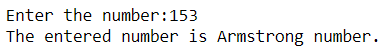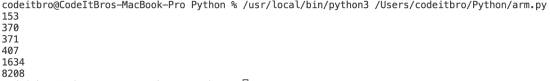# Python Program to Check Armstrong Number [In an Interval]

2141

Today, we will see a simple Python 3 program to check Armstrong’s number. If you missed our previous Python 3 blog, check it out, where I have created a simple Python calculator.

To understand this example, you should know the following Python programming concepts.

• Variables and Datatypes
• If-else statement
• While loop

You can also refer to these best Python books for beginners to add more resources to your learning path.

We will soon cover these topics in detail in our Python introductory course. Subscribe to our mailing list to get all the latest updates about the course straight to your inbox.

## Python Program to Check Armstrong Number

A number is called an Armstrong number when the number is equal to the sum of its digits raised to the total number of digits.

For example, let’s check if 153 is an Armstrong number or not. Here, the total number of digits is 3. Therefore, we will calculate the cubes of individual figures and see if their sum equals the number itself.

Cube of 1 = 1
Cube of 5  = 125
Cube of 3 = 27

Now,

Cube of 1 + Cube of 5 + Cube of 3 = 153 (the number itself). Hence, 153 is an Armstrong number.

In our Python code, we have to replicate the same logic to check whether the given number is an Armstrong number or not.

Step 1: Get the input from the user to check.

Step 2: Store the input in a temporary variable.

Step 3: Create a variable to store the sum and initiate it with zero.

Step 4: Now, find the length of the input number by using the len() function and store it in a variable.

Step 5: Use the While loop to find the sum of individual digits raised to the length of the number.

Step 6: Use the if-else statement to check if the sum is equal to the actual or not. If it is equal, then the user will see the message that the entered number is Armstrong; otherwise, the number is not an Armstrong number.

### Source Code:

```num = int(input("Enter the number:"))
temp = num
sum = 0
power = len(str(num))
while temp > 0:
digit = temp % 10
sum = sum + digit ** power
temp = temp // 10
if num == sum:
print("The entered number is Armstrong number.")
else:
print("Entered number is not a armstrong number")```

### Program Output:

Enter the number: 153
The entered number is Armstrong number.## Python Program to Check Armstrong Number In An Interval

Code:

```#Python program to print armstrong numbers in an interval

LowerNum = 3000
UpperNum = 5000
for i in range(LowerNum, UpperNum + 1):

order = len(str(i))

sum = 0
temp = i
while temp > 0:
digit = temp % 10
sum += digit ** order
temp //= 10

if i == sum:
print(i)```

Output: